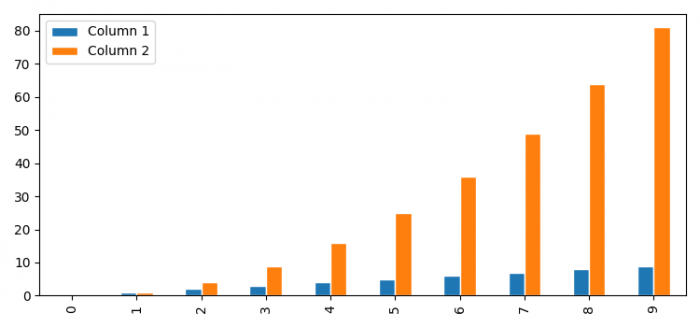# How to change the space between bars when drawing multiple barplots in Pandas? (Matplotlib)

To change the space between bars when drawing multiple barplots in Pandas within a group, we can use linewidth in plot() method.

## Steps

• Set the figure size and adjust the padding between and around the subplots.
• Make a dictionary with two columns.
• Create a two-dimensional, size-mutable, potentially heterogeneous tabular data.
• Plot the dataframe with plot() method, with linewidth that change the space between the bars.
• Place a legend on the plot.
• To display the figure, use show() method.

## Example

import pandas as pd
from matplotlib import pyplot as plt

plt.rcParams["figure.figsize"] = [7.50, 3.50]
plt.rcParams["figure.autolayout"] = True

d = {'Column 1': [i for i in range(10)],
'Column 2': [i * i for i in range(10)]}

df = pd.DataFrame(d)
df.plot(kind='bar', edgecolor='white', linewidth=1)

plt.legend(loc="upper left")
plt.show()

## Output